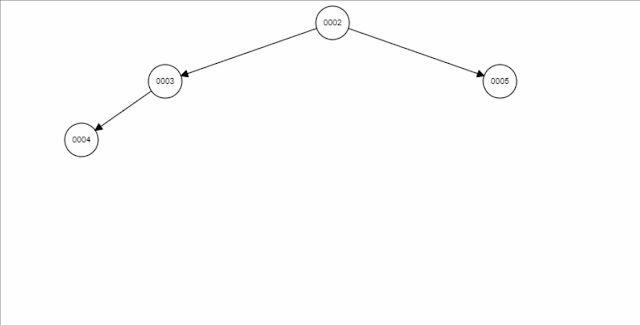# Binary Heap -Min and Max heap using Typescript

## Binary heap

The binary heap was introduced by J. W. J. Williams in 1964, as a data structure for heapsort.

A binary heap is a special kind of a heap data structure that satisfies the heapsort property. It is a tree-like structure with two children nodes, one on the left and one on the right.

In this section we are going to learn how to implement Min Heap and Max Heap using Typescript. Min-heap lowest item is always on the root, while in the max-heap highest value is on the root. Both can use both to implement a priority queue. Some languages have in-built heap implementations, but some languages like C# and JavaScript do not have, but we can easily create our own.

A heap implemented with a binary tree that follows the following two rules:

• Each node’s Element is greater than or equal to the elements of that node’s children…
• The tree is a complete binary tree.

## How heap store the data

Heaps, like binary search trees, do not use pointers to store their children. The heap stored item in the array, but its operations are more easily understood by looking at the binary tree representation. There is no ambiguity in the mapping between the array representation and the binary tree representation. To obtain the array, you can traverse the tree in level order.

We will discuss the prevalent data structure `MinHeap`. Minheap is also used to implement a priority queue. There are two types of the heap.

• MinHeap - It stores the min value at the root
• MaxHeap- It stores the max value at the root

This blog will discuss how to implement min heap in typescript(javascript). Many languages provide heap implementation in their framework, but some do not have inbuilt heaps like C# and JavaScript.

We want to insert a node with value 01 to the heap on the left.## Extract Min Element``````export class MinHeap {
private items: Array<number>;
constructor() {
this.items = [];
}
this.items.push(item);
this.heapifyUp(this.items.length - 1);
}
public extractMin() {
if (this.count > 0) {
let item = this.items;
this.items = this.items.pop();
this.heapifyDown(0);
return item;
}
return Infinity;
}
public get count() {
return this.items.length;
}
private heapifyUp(index: number) {
let parent = this.parent(index);
if (parent >= 0 && this.items[parent] > this.items[index]) {
this.swap(this.items, parent, index);
this.heapifyUp(parent);
}
}
private heapifyDown(index: number) {
let smallest = index;
let leftChild = this.letChild(index);
let rightChild = this.rightChild(index);
if (leftChild < this.count && this.items[leftChild] < this.items[index]) {
smallest = leftChild;
}
if (
rightChild < this.count &&
this.items[rightChild] < this.items[smallest]
) {
smallest = rightChild;
}
if (smallest != index) {
this.swap(this.items, index, smallest);
this.heapifyDown(smallest);
}
}
private parent(index: number): number {
if (index < 0) return -1;
return Math.floor((index - 1) / 2);
}
private letChild(index: number) {
if (index > 0) {
return Math.floor((2 * index + 1) / 2);
}
return undefined;
}
private rightChild(index: number) {
if (index > 0) {
return Math.floor((2 * index + 2) / 2);
}
return undefined;
}
private swap(arr: Array<number>, i: number, j: number) {
let temp = arr[i];
arr[i] = arr[j];
arr[j] = temp;
}
}

``````

## Interview Questions

1.https://gist.github.com/knyl2013/1534d0319e1689bdea20ed7963803ba1

Fixed the indexing issues and made the min heap generic (can use types beside just numbers)

1.Thanks for the fix :)